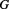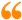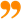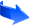﻿ Mass-Generation Mechanism Based on the Duality of Time Theory## Mass-Generation Mechanism Based on the Duality of Time Theory

Yang-Mills fields are gauge fields used in modern physics to describe physical fields that play the role of carriers in fundamental interaction. In 1954, C.N. Yang and R.L. Mills suggested that the space of intrinsic degrees of freedom of elementary particles depends on the points of space-time, and the intrinsic spaces corresponding to different points are not canonically isomorphic. In geometrical terms, this suggestion means that the space of intrinsic degrees of freedom is a vector bundle over space-time that does not have a canonical trivialization, and physical fields are described by cross-sections of this bundle. To describe the differential evolution equation of a field one has to define a connection in the bundle, that is, a trivialization of the bundle along the curves in the base. Such a connection with a fixed holonomy group describes a physical field, usually called a Yang-Mills field. The equations for a free Yang-Mills field can be deduced from a variational principle. They are a natural non-linear generalization of Maxwell’s equations.

The most important Quantum Field Theories describing elementary particles are gauge theories formulated in terms of a principal fibre bundle over the Minkowski space-time with particular choices of the structure group. Given an arbitrary compact gauge group, does a non-trivial quantum Yang-Mills theory with a finite mass gap exist?

This problem is listed as one of the Millennium Prize Problems in mathematics given by the Clay Institute, which is to prove that for any compact simple gauge group, a non-trivial quantum Yang–Mills theory exists onand has a mass gap. Existence includes establishing strong axiomatic properties, such as those cited in Streater & Wightman (1964), Osterwalder & Schrader (1973) and Osterwalder & Schrader (1975).

As it was accurately anticipated by Jaffe and Witten in the Official Problem Description, that establishing the existence of Yang-Mills theory and mass gap requires the introduction of fundamental new ideas both in physics and in mathematics. Hence, the innovative concept of the Duality of Time and perpetual creation of space provide a dynamic granular structure of space-time which clearly indicates the existence of a minimum physical mass as a result of the coupling between at least two geometrical points, or the individual monads that are the temporal images of the only one Single Monad that is actually described by real existence at the real flow of time. Since the massless monads are abruptly bursting from vacuum to the speed of light, and because they cannot coexist together in the inner level of time, when two monads couples to form a physical particle their minimum average velocity on the normal outward level of time would equal at least half the speed of light, because the speed of each one of them must be either zero or, which gives the particle a minimum positive energy or rest mass.

The problem in most current theories is that they consider space-time as a background continuum, where particles propagate in continuous existence, which means that they can acquire any infinitesimal energy. With the new concept of the Duality of Time and perpetual creation of space, there is no motion in the common sense that the object or particle gradually leaves its place to occupy new infinitesimally adjacent places, but it is perpetually re-created in those new places. Therefore, there is no instantaneous transmutation but an average change between the discrete points of space, which also takes a discrete duration of time. These discrete instances of space-time are created dynamically to form the aether which then may condense into matter particles at some higher excited states. This dynamic aether does not contradict the null result of Michelson-Morley experiment which proved that light travels with the same speed regardless whether it was moving in the direction of the movement of the Earth or perpendicular to it. On the contrary, this constancy and invariance of the speed of light can now be explained as the speed of the dynamic creation of space, and it is the only real speed in nature, while the limited velocities of objects and matter particles are a result of averaging over the inner levels of time.

It is well established in modern physics that mass is an emergent property, and since the Standard Model relies on gauge and chiral symmetry, the observed non-zero masses of elementary particles require spontaneous symmetry breaking, which suggested the existence of the massive Higgs boson, whose own mass is not explained in the model. This Higgs mechanism is part of the Glashow-Weinberg-Salam theory to unify electromagnetic and weak interactions.

However, the ground state of matter according to the Duality of Time Theory is a perfect super fluid, where masses of elementary particles could arise from interaction with the physical vacuum, in a manner similar to the gap generation mechanism.

Moreover, the Duality of Time Theory provides an even more fundamental and very simple mechanism for mass generation, in full agreement with the principles of Classical Mechanics.

In general, the fundamental reason for inertial mass is the coupling between the particles that constitute the object, because the binding field enforces specific separations between them, so that when the position of one particle changes, a finite time elapses before other particles move, due to the finite speed of light. This delay is the cause of inertial behavior, and this implies that all massive particles are composed of more sub-particles, and so on until we reach the most fundamental particles which should be massless.

This description is (and can only be) fulfilled by the Duality of Time Theory, due to the discrete symmetry of the genuinely-complex time-time geometry as described in the Duality of Time Theory is based on the Single Monad Model, so the fundamental reason of the granular geometry is the fact that no two geometrical points can exist at the same real instance of time, so they must be re-created in one chronological sequence.

This delay is what causes the inertial mass, so physical objects are dynamically formed by the coupling between at least two geometrical points which produces the entangled dimensions. According to the different degrees of freedom in the resulting spatial dimensions, this entanglement is responsible for the various coupling parameters, including charge and mass, which become necessarily quantized because they are proportional to the number of geometrical nodes constituting each state, starting from one individual point for massless bosons.

Nevertheless, some bosons might still appear to have heavy masses (in our outer level of time) because they are confined in their lower dimensions in which they are massless, just as the inertial mass of normal objects is exhibited only when they are moved in the outer level of time. Consequently, there is a minimum mass gap above the ground state of vacuum (c, 0), which is itself also above the void state (0, 0). This is because each single geometrical node is massless on its own dimensions, while the minimum state above this ground state is composed of two nodes which must have non-zero inertial mass because of the time delay between their sequential creation instances.

This important conclusion agrees with Yang-Mills suggestion that the space of intrinsic degrees of freedom of elementary particles depends on the points of space-time. It was already anticipated that proving Yang-Mills conjecture requires the introduction of fundamental new ideas both in physics and in mathematics. Accordingly, due to the sequential re-creation in the inner levels of time, the Duality of Time Theory introduced the genuinely-complex time-time geometry which revealed this profound discrete symmetry that makes the vacuum physical and dynamic, with super fluid properties and discrete mass states.

### List of Some Major Problems Solved by the Duality of Time Theory

In the following articles, we will list some of the major unsolved problems in theoretical physics and describe them in brief, stating their potential solutions according to the Duality of Time Postulate. Although many of these problems will be simply eliminated according to the new genuinely-complex time-time geometry, a detailed theoretical and mathematical analysis is required in order to explain how these problems are settled. Therefore, some of the following brief suggested solutions may be speculative.

These articles are extracted from Chapter III of the Duality of Time book, and some of the details are discussed further in other chapters, as well as Volume III that is the Ultimate Symmetry. A more concise description is also published in Time Chest.

### The Duality of Time PostulateTo understand how all these major problems could be solved so easily, please study first the Duality of Time Postulate, that has been extracted from Chapter V of the Duality of Time book.

### Message from the Author:I have no doubt that this is the most significant discovery in the history of mathematics, physics and philosophy, ever!

This unique understanding of geometry will cause a paradigm shift in our knowledge of the fundamental nature of the cosmos and its corporeal and incorporeal structures.

Enjoy reading... , all the Best !

Mohamed bin Ali Haj Yousef

Check this detailed video presentation on "Deriving the Principles of Special, General and Quantum Relativity Based on the Single Monad Model Cosmos and Duality of Time Theory".The science of Time is a noble science, that reveals the secret of Eternity. Only the Elites of Sages may ever come to know this secret. It is called the First Age, or the Age of ages, from which time is emerging.Ibn al-Arabi [The Meccan Revelations: Volume I, page 156. - Trns. Mohamed Haj Yousef]

### Ibn al-Arabi Website:### The Meccan Revelations:### The Sun from the West:## Social SharingLike Our Facebook Page:Like Our Facebook Page:Moonlight floods the whole sky from horizon to horizon. How much it can fill your room, depends on the windows !